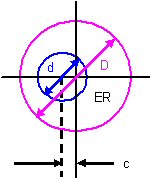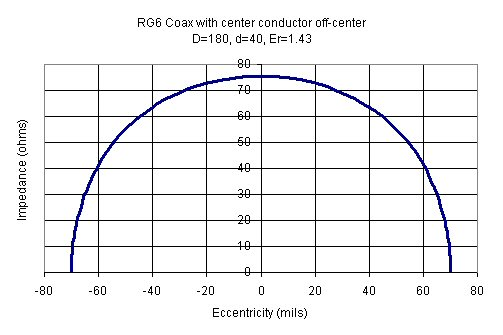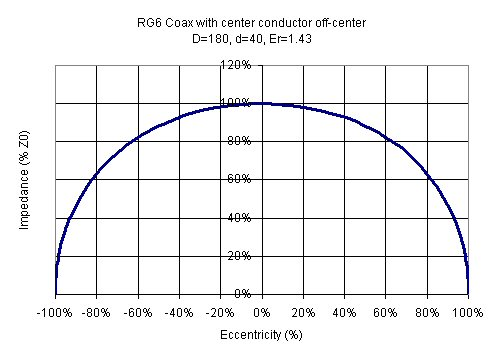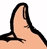# Off-Center Coax

This is a new page for December 2006! Someone recently asked us for an equation for calculating the characteristic impedance of off-center coax. Here it is:

For outer diameter D, inner diameter d, and eccentricity c:

Z0=[60/sqrt(ER)] x acosh[(1/2)x(D/d+d/D-4c^2/(dxD)]We entered the equation into Excel, and sure enough, if c=0 you get the same exact impedance you get from the "normal" equation for coax:

Z0=[60/sqrt[ER]) x ln(D/d)

The above equation is slightly more accurate this form which seems to be more popular:

Z0=[138/sqrt[ER]) x log(D/d)

We'll accept that as strong evidence that the off-center equation is correct.

Why might a coax cable have the center conductor eccentric to the outer conductor? Every time you bend a cable, you're probably disturbing the center conductor from its preferred position!

Next we used RG6 to provide and example. RG6 is cheap 75 ohm coax cable you use to hook up your Dish Network. The center conductor is approximately 40 mils, while the inside diameter of the outer conductor is 180 mils. Therefore the radial thickness of the dielectric is 70 mils. This puts a boundary on the maximum eccentricity (70 mils) otherwise the two conductors collide!

Below is a plot of the impedance versus eccentricity. You'll notice you'd have to be off by a full 55 mils before the 75 ohms impedance drops to 50 ohms! Also note that when you hit the maximum eccentricity of 70 mils the impedance goes to zero, which is another intuitive bit of evidence that the off-center equation is correct.Let's look at the response with normalized coordinates. Eccentricity can be plotted in percent, in this case 70 mils would be 100% eccentric. That fancy acosh function sure looks like a semicircle to us! Maybe someday when we're bored we'll try to fit X^2+Y^2=R^2 to see the difference. Notice that a fifty percent concentricity error results in a ten percent impedance error.So what's the bottom line? Unless you need extremely accurate cable impedance, a little eccentricity in aligning the center conductor won't make a huge difference.

Time for a Microwaves101 rule of thumb!Coax line impedance is not a strong function of the eccentricity of the center conductor. You can be off by a full 50% and the impedance will decrease on the order of only 10%! And remember, impedance can only decrease if the center conductor is off center, it will never increase!

Author : Unknown Editor